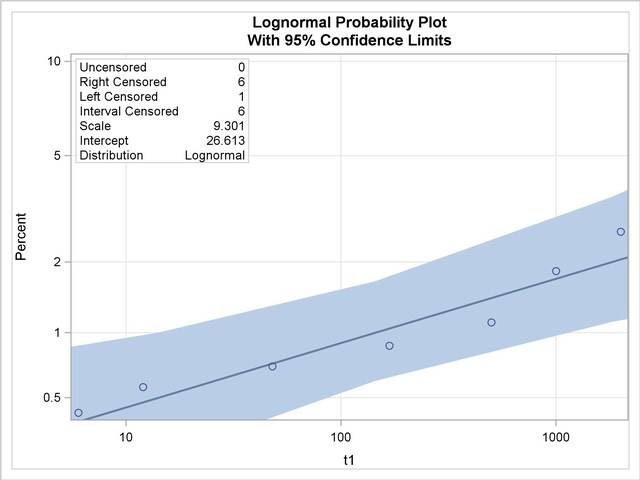The LIFEREG Procedure

## Example 48.6 Probability Plotting—Arbitrary Censoring

Table 48.7 contains microprocessor failure data (Nelson; 1990). Units were inspected at predetermined time intervals. The data consist of inspection interval endpoints (in hours) and the number of units failing in each interval. A missing (.) lower endpoint indicates left censoring, and a missing upper endpoint indicates right censoring. These can be thought of as semi-infinite intervals with a lower (upper) endpoint of negative (positive) infinity for left (right) censoring.

Table 48.7 Interval-Censored Data

Lower Endpoint

Upper Endpoint

Number Failed

.

6

6

6

12

2

24

48

2

24

.

1

48

168

1

48

.

839

168

500

1

168

.

150

500

1000

2

500

.

149

1000

2000

1

1000

.

147

2000

.

122

The following SAS statements create the SAS data set Micro:

```   data Micro;
input t1 t2 f ;
datalines;
. 6 6
6 12 2
12 24 0
24 48 2
24 .  1
48 168 1
48 .   839
168 500 1
168 .   150
500 1000 2
500 .    149
1000 2000 1
1000 . 147
2000 . 122
;
run;
```

The following SAS statements compute the nonparametric Turnbull estimate of the cumulative distribution function and create a lognormal probability plot:

```   ods graphics on;
proc lifereg data=Micro;
model ( t1 t2 ) = / d=lognormal intercept=25 scale=5;
weight f;
probplot
pupper = 10
itprintem
printprobs
maxitem = (1000,25)
ppout;
inset;
run;
ods graphics off;
```

The two initial values INTERCEPT=25 and SCALE=5 in the MODEL statement are used to aid convergence in the model-fitting algorithm.

The following tables are created by the PROBPLOT statement in addition to the standard tabular output from the MODEL statement. Output 48.6.1 shows the iteration history for the Turnbull estimate of the CDF for the microprocessor data. With both options ITPRINTEM and PRINTPROBS specified in the PROBPLOT statement, this table contains the log likelihoods and interval probabilities for every 25th iteration and the last iteration. It would contain only the log likelihoods if the option PRINTPROBS were not specified.

Output 48.6.1 Iteration History for the Turnbull Estimate
The LIFEREG Procedure

Iteration History for the Turnbull Estimate of the CDF
Iteration Loglikelihood (., 6) (6, 12) (24, 48) (48, 168) (168, 500) (500, 1000) (1000, 2000) (2000, .)
0 -1133.4051 0.125 0.125 0.125 0.125 0.125 0.125 0.125 0.125
25 -104.16622 0.00421644 0.00140548 0.00140648 0.00173338 0.00237846 0.00846094 0.04565407 0.93474475
50 -101.15151 0.00421644 0.00140548 0.00140648 0.00173293 0.00234891 0.00727679 0.01174486 0.96986811
75 -101.06641 0.00421644 0.00140548 0.00140648 0.00173293 0.00234891 0.00727127 0.00835638 0.9732621
100 -101.06534 0.00421644 0.00140548 0.00140648 0.00173293 0.00234891 0.00727125 0.00801814 0.97360037
125 -101.06533 0.00421644 0.00140548 0.00140648 0.00173293 0.00234891 0.00727125 0.00798438 0.97363413
130 -101.06533 0.00421644 0.00140548 0.00140648 0.00173293 0.00234891 0.00727125 0.007983 0.97363551

The table in Output 48.6.2 summarizes the Turnbull estimates of the interval probabilities, the reduced gradients, and Lagrange multipliers as described in the section Arbitrarily Censored Data.

Output 48.6.2 Summary for the Turnbull Algorithm
. 6 0.0042 0 0
6 12 0.0014 0 0
24 48 0.0014 0 0
48 168 0.0017 0 0
168 500 0.0023 0 0
500 1000 0.0073 -7.219342E-9 0
1000 2000 0.0080 -0.037063236 0
2000 . 0.9736 0.0003038877 0

Output 48.6.3 shows the final estimate of the CDF, along with standard errors and nonparametric confidence limits. Two kinds of nonparametric confidence limits, pointwise or simultaneous, are available. The default is the pointwise nonparametric confidence limits. You can specify the simultaneous nonparametric confidence limits by using the NPINTERVALS=SIMUL option.

Output 48.6.3 Final CDF Estimates for Turnbull Algorithm
Cumulative Probability Estimates
Probability
Pointwise 95% Confidence
Limits
Standard Error
Lower Upper
6 6 0.0042 0.0019 0.0094 0.0017
12 24 0.0056 0.0028 0.0112 0.0020
48 48 0.0070 0.0038 0.0130 0.0022
168 168 0.0088 0.0047 0.0164 0.0028
500 500 0.0111 0.0058 0.0211 0.0037
1000 1000 0.0184 0.0094 0.0357 0.0063
2000 2000 0.0264 0.0124 0.0553 0.0101

Output 48.6.4 shows the CDF estimates, maximum likelihood fit, and pointwise parametric confidence limits plotted on a lognormal probability plot.

Output 48.6.4 Lognormal Probability Plot for the Microprocessor DataPrevious Page | Next Page | Top of Page